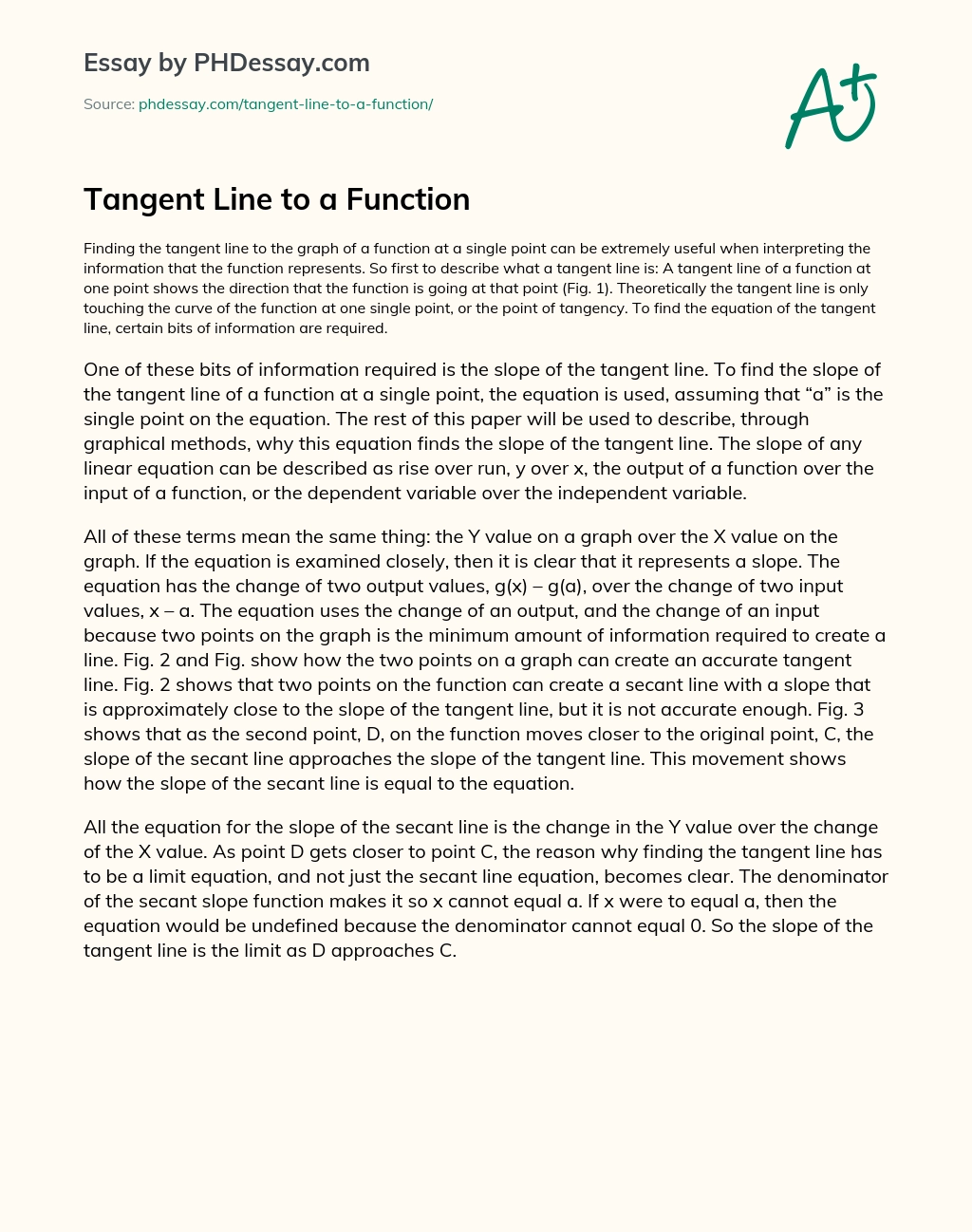Last Updated 05 Aug 2021

# Tangent Line to a Function

Essay type Research
Words 376 (1 page)
Views 188

Finding the tangent line to the graph of a function at a single point can be extremely useful when interpreting the information that the function represents. So first to describe what a tangent line is: A tangent line of a function at one point shows the direction that the function is going at that point. Theoretically the tangent line is only touching the curve of the function at one single point, or the point of tangency. To find the equation of the tangent line, certain bits of information are required.

One of these bits of information required is the slope of the tangent line. To find the slope of the tangent line of a function at a single point, the equation is used, assuming that “a” is the single point on the equation. The rest of this paper will be used to describe, through graphical methods, why this equation finds the slope of the tangent line. The slope of any linear equation can be described as rise over run, y over x, the output of a function over the input of a function, or the dependent variable over the independent variable.

All of these terms mean the same thing: the Y value on a graph over the X value on the graph. If the equation is examined closely, then it is clear that it represents a slope. The equation has the change of two output values, g(x) – g(a), over the change of two input values, x – a. The equation uses the change of an output, and the change of an input because two points on the graph is the minimum amount of information required to create a line.

Order custom essay Tangent Line to a Function with free plagiarism report

GET ORIGINAL PAPER

All the equation for the slope of the secant line is the change in the Y value over the change of the X value. As point D gets closer to point C, the reason why finding the tangent line has to be a limit equation, and not just the secant line equation, becomes clear. The denominator of the secant slope function makes it so x cannot equal a. If x were to equal a, then the equation would be undefined because the denominator cannot equal 0. So the slope of the tangent line is the limit as D approaches C.This essay was written by a fellow student. You can use it as an example when writing your own essay or use it as a source, but you need cite it.

## Get professional help and free up your time for more important courses

Starting from 3 hours delivery 450+ experts on 30 subjects
get essay help 124  experts online

Did you know that we have over 70,000 essays on 3,000 topics in our database?